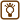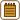## Unknown Nitrogen Oxide

### Task number: 1798

In a vessel, whose volume is 1 l, there is a gas, which is a compound of oxygen and nitrogen. The gas’s mass is 1 g, gas’s temperature is 17 °C and pressure of the gas is 31.7 kPa. Determine the chemical formula and name of the compound.

• #### Hint

Using the ideal gas law, determine the molar mass of the unknown gas first.

Then find such a combination of the number of oxygen and nitrogen atoms in the molecule, so that th sum of molar masses of these atoms corresponds to the found molar mass of the gas.

• #### Notation

 V = 1 l = 10-3 m3 volume of the gas m = 1 g = 10-3 kg mass of the gas t = 17 °C ⇒ T = 290 K temperature of the gas p = 31.7 kPa = 31.7·103 Pa pressure of the gas NxOy  x = ?  y = ? unknown formula of the gas

From the Handbook of Chemistry and Physics:

 R = 8.31 J K-1 mol-1 molar gas constant Ar(N) = 14 relative atomic mass of nitrogen N Ar(O) = 16 relative atomic mass of oxygen O
• #### Analysis

From the task assignment we know all state quantities of the unknown gas that we need for the ideal gas law. Let us assume that properties of the unknown gas are not so different from the properties of an ideal gas. We can determine the unknown gas’s molar mass from the equation of state.

Molar mass in g mol-1 is numerically equal to the relative molecular mass, which could by determined as the sum of the relative atomic masses of the atoms that the molecule is composed of. Hence we determine the molecule structure of the unknown gas.

• #### Solution

We use the ideal gas law

$pV\,=\,nRT\,\mathrm{,}$

into which we substitute the unknown amount of substance n expressed by the total mass of the gas m and its molar mass Mm:

$n\,=\,\frac{m}{M_m}\,.$

From the ideal gas law, we express the unknown molar mass Mm and substitute given values into obtained equation:

$pV\,=\,\frac{m}{M_m}RT\,\mathrm{,}$ $M_m\,=\,\frac{mRT}{pV}\,=\,\frac{10^{-3}\cdot 8{.}31\cdot{290}}{31{.}7\cdot{10^3}\cdot{10^{-3}}}\,\mathrm{kg\,mol^{-1}}$ $M_m\,\dot=\,0{.}076\,\mathrm{kg\,mol^{-1}}\,=\,76\,\mathrm{g\,mol^{-1}}.$

Hence it follows that the relative molar mass of the compound is 76 and it holds that:

$A_r\left(\mathrm{N}_x\mathrm{O}_y\right)\,=\,xA_R\left(\mathrm{N}\right)\,+\,yA_R\left(\mathrm{O}\right)$ $76\,=\,14x\,+\,16y.$

where x and y have to be small natural numbers.

Thus let us try to compute the value of y for values of x:

$x\,=\,1\hspace{30px}y\,=\,3{.}88$ $x\,=\,2\hspace{30px}y\,=\,3$ $x\,=\,3\hspace{30px}y\,=\,2{.}13$ $x\,=\,4\hspace{30px}y\,=\,1{.}25$

From this summary we see, that the compound of two nitrogen atoms and three oxygen atoms, i.e. dinitrogen trioxide N2O3, suits the relative molecular mass the best.

• #### Answer

The unknown compound is dinitrogen trioxide N2O3.